[动力学][有限元]模态分析基础 (Basics of Modal Analysis) (《有限单元法-编程与软件应用》章节节选)

《有限单元法：编程与软件应用》模态分析章节的内容节选：

• 基本方程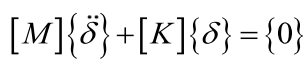(10.1‑1)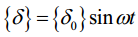(10.1‑2)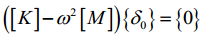(10.1‑3)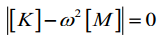(10.1‑4)

• 求解方法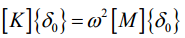(10.1‑5)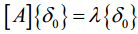(10.1‑6)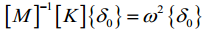(10.1‑7)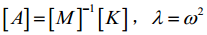[K]-1存在，则公式变为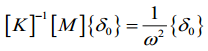(10.1‑8)。

• 相关内容 ( Related Topics )

.

. 2D Truss by Matlab [Matlab 2D桁架有限元] (code by myself)

. 3D Truss by MATLAB[MATLAB 3D 桁架静力分析] (code by myself)

. [FEM][有限元][编程][Matlab][Code by myself] FEM Analysis: 2D Truss Element [有限元分析: 2D桁架单元]

. [FEM][有限元][编程][Matlab][Code by myself] FEM Analysis: 2D Euler Beam Element [有限元分析: 2D欧拉梁单元]

. [FEM][有限元][编程][Matlab][Code by myself] 2D剪切梁单元

. [FEM][有限元][编程][Matlab][Code by myself] 2D Timoshenko梁单元

. [FEM][有限元][编程][Matlab][Code by myself] 三角形常应变单元(CST)

. [FEM][有限元][编程][Matlab][Code by myself] 平面4变形单元(Q4)

. [FEM][有限元][编程][Matlab][Code by myself] 4节点四面体单元(TET4)

. [FEM][有限元][编程][Matlab][Code by myself] 8节点六面体单元(C3D8)

. [FEM][有限元][编程][Matlab][Code by myself] 平面8节点二次“完全积分”单元(CPS8)

. [FEM][有限元][编程][Matlab][Code by myself] 平面6节点二次“完全积分”单元(CPS6)

. [FEM][MATLAB][有限元] FEM Modal Analysis Programming with MATLAB (Truss Element) (桁架单元模态分析编程)

. [FEM][MATLAB][有限元] FEM Modal Analysis Programming with MATLAB (Frame Elements) (框架单元模态分析编程)

. [力学][有限元]Basics of Linear Buckling Analysis [线性曲屈分析基础]

. [FEM][MATLAB][有限元][编程] FEM Buckling Analysis Programming with MATLAB (Truss Elements) (桁架单元曲屈分析编程)

. [FEM][MATLAB][有限元][编程] FEM Buckling Analysis Programming with MATLAB (Frame Elements) (框架单元曲屈分析编程)

5 1

( 如果您发现有错误，欢迎批评指正。邮箱：jidong_cui@163.com . 如果您喜欢这篇博文，请在上面给我 点个赞 吧！( If you found any mistakes in the post, please let me know. Email : jidong_cui@163.com. If you like this posts, please give me a thumbs up rating on the above button! )

• 微信公众号 ( Wechat Subscription)2 thoughts on “[动力学][有限元]模态分析基础 (Basics of Modal Analysis) (《有限单元法-编程与软件应用》章节节选)”

This site uses Akismet to reduce spam. Learn how your comment data is processed.